# Longest Common Subsequence

Suppose you and I each had an ordered list of items and we were interested in comparing how similar those lists are. One calculation we can perform on these two strings is the Longest Common Subsequence. A sequence X is an ordered list of elements <x1, …, xn>. A subsequence Z is another sequence where (1) Each element of Z is also an element of X and (2) The elements of Z occur in the same order (in Z) as they do in X.

Note that we do not say that the elements of Z need to be a continuous block of elements. If this were true we would be defining a substring. So as an example, suppose we have as an initial string,
X = C, O, M, P, U, T, E, R.
Then the following are all subsequences:
Z1 = C, M, U, T, R
Z2 = C, O, M, P
Z3 = U, T, E, R
Z4 = O, P, T, E

I will note that Z2 and Z3 are also substrings since they contain continuous sets of characters.

The length of a substring is simply the number of characters it contains. So X has length 8, Z1 has length 5, Z2, Z3 and Z4 have length 4.

Suppose now that we had a second string, Y = P, R, O, G, R, A, M, M, E, R and are interested in the longest common subsequence between the two. We can do that by observing that there is a bit of recursion going on with this question. What I mean by that is that asking the question of “What is the longest common subsequence between X and Y” is the same as asking “What is the longest common subsequence between X and Y once we have seen 8 characters of X and 10 characters of Y”

There are three possible ways to answer this question.

If X<sub>8</sub> is not equal to Y<sub>10</sub>, then the answer to this will be the same as the maximum of the pair X<sub>7</sub>, Y<sub>10</sub> and the pair X<sub>8</sub>, Y<sub>9</sub>.
If we reach a situation where we reach the beginning of either string, we are forced to answer 0 to that question.

Then the function has the following look:

LCS(Xi, Yj) =
 0, if i is 0 or j is 0 1 + LCS(Xi-1, Yj-1) if Xi equals Yj max(LCS(Xi-1, Yj), LCS(Xi, Yj-1))

Below is a table showing how we would solve the problem mentioned.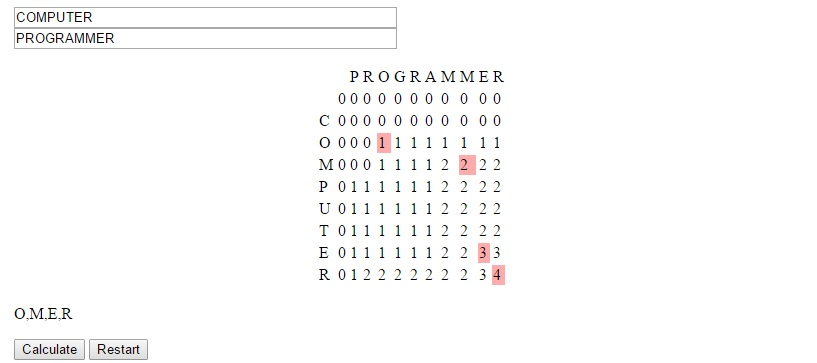The strategy used to devise this concept is called dynamic programming. It is useful we can solve larger problems by solving overlapping subproblems, as was the case here. In this situation we generally can store the data in a table form and avoid re-solving subproblems for which many larger problems will be dependent.

You can see this algorithm in action at LEARNINGlover.com: Longest Common Subsequece. Let me know what you think.

# Lets Learn About XOR Encryption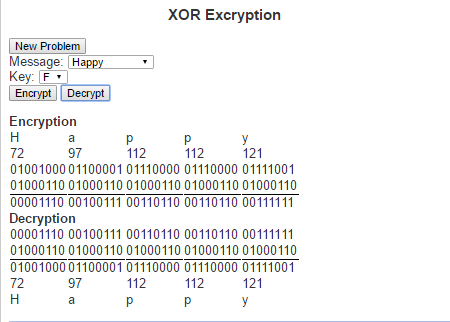One of the more common things about this generation is the constant desire to write up (type) their thoughts. So many of the conversations from my high school days were long lasting, but quickly forgotten. Today’s generation is much more likely to blog, tweet, write status updates or simply open up a notepad file and write up their thoughts after such a conversation.

When we feel that our thoughts are not ready for public eyes (maybe you want to run your idea by the Patent and Trademark Office before speaking about it) we may seek some form of security to ensure that they stay private. An old fashioned way of doing this was to write in a diary and enclosed it within a lock and key. The mathematical field of encryption also tries to grant privacy by encoding messages so that only people with the necessary information can read them.

The type of encryption I want to speak about today is called XOR encryption. It is based on the logical operation called “exclusive or” (hence the name XOR). The exclusive or operation is true between two logical statements if exactly one of the two statements is true, but not both statement. This can be represented with the following truth table

 Input 1 Input2 XOR Result T T F T F T F T T F F F

XOR Encryption is particularly useful in this day and age because we every character we type is understood by the computer as a sequence of zeros and ones. The current standard encoding that is used is Unicode (also known as UTF-8). Under this encoding the letter ‘a’ is represented as the binary string ‘01100001’. Similarly every letter, number and special character can be represented as its own binary string. These binary strings are just an assignment of numbers to these characters so that we can to help represent them in the computer. The numbers can the be thought of in base 10, which is how we generally think about numbers, or in base 2 which is how computers generally work with numbers (or a number of other ways). The way we would use these binary strings in encoding is first by translating a text from human-readable text to machine readable text via its binary string. For example, the word “Invincible”, we would get the following binary strings:

 Letter Unicode in base 10 Unicode in base 2 I 73 01001001 n 110 01101110 v 118 01110110 i 105 01101001 n 110 01101110 c 99 01100011 i 105 01101001 b 98 01100010 l 108 01101100 e 101 01100101

To encrypt the message we need a key to encode the message and will simply perform an XOR operation on the key and every character in the string. Similarly, do decrypt the message we perform XOR operation on the key and every character in the encoded message. This means that the key (much like a normal key to a diary) must be kept private and only those whom the message is to be shared between have access to it.

Here is a link to the script where you can check out XOR Encrpytio. Try it out and let me know what you think.

# Topological Sort

One of the things I generally say about myself is that I love learning. I can spend hours upon hours reading papers and algorithms to better understand a topic. Some of these topics are stand alone segments that I can understand in one sitting. Sometimes, however, there is a need to read up on some preliminary work in order to fully understand a concept.

Lets say that I was interested in organizing this information into a new course. The order I present these topics is very important. Knowing which topics depend on one another allows me to use the topological sorting algorithm to determine an ordering for the topics that respects the preliminary work.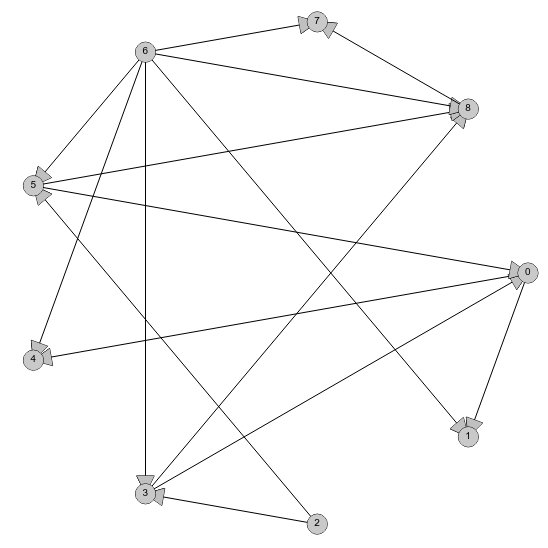The input for the topoligical sorting algorithm is a Directed Acyclic Graph (DAG). This is a set of relationships between pairs of topics, where if topic 1 must be understood before topic 2, we would add the relationship (topic 1, topic 2) to the graph. DAGs can be visualized by a set of nodes (points) representing the topics. Relationships like the one above (topic 1, topic 2) can then represented by a directed arc originating at topic 1 and flowing in the direction of topic 2. We say that the graph is “Acyclic” because there cannot be a cycle in the topic preliminaries. This amounts to us saying that a topic cannot be a prerequisite for itself. An example of a DAG is shown in the image above.

With the topics represented as a DAG, the topologial ordering algorithm works by searching the set of nodes for the one with no arcs coming into it. This node (or these nodes is multiple are present) represents the topic that can be covered next without losing understanding of the material. Such a node is guaranteed to exist by the acyclic property of the DAG. Once the node is selected, we can remove this node as well as all arcs that originate at this node from the DAG. The algorithm then repeats the procedure of searching for a nod with no arcs coming into it. This process repeats until there are no remaining nodes from which to choose.

Now lets see how the topological sort algorithm works on the graph above. We will first need to count the in-degree (the number of arcs coming into) each node.

Node | Indegree
—————-
0 | 2
1 | 2
2 | 0
3 | 2
4 | 2
5 | 2
6 | 0
7 | 2
8 | 3

Node to be removed (i.e. node with the minimum indegree): Node 2.
Arcs connected to node 2: (2, 5), (2, 3)
Resulting Indegree Count:
Node | Indegree
—————-
0 | 2
1 | 2
3 | 1
4 | 2
5 | 1
6 | 0
7 | 2
8 | 3

Node to be removed: Node 6:
Arcs connected to node 6: (6, 1), (6, 3), (6, 4), (6, 5), (6, 7), (6, 8)
Resulting Indegree Count:
Node | Indegree
—————-
0 | 2
1 | 1
3 | 0
4 | 1
5 | 0
7 | 1
8 | 2

Node to be removed: Node 3
Arcs connected to node 3: (3, 0), (3, 8)
Resulting Indegree Count:
Node | Indegree
—————-
0 | 1
1 | 1
4 | 1
5 | 0
7 | 1
8 | 1

Node to be removed: Node 5
Arcs connected to node 5: (5, 0), (5, 8)
Resulting Indegree Count:
Node | Indegree
—————-
0 | 0
1 | 1
4 | 1
7 | 1
8 | 0

Node to be removed: Node 0:
Arcs connected to node 0: (0, 1), (0, 4)
Resulting Indegree Count:
Node | Indegree
—————-
1 | 0
4 | 0
7 | 1
8 | 0

Node to be removed: Node 1
Arcs connected to node 1: none
Resulting Indegree Count:
Node | Indegree
—————-
4 | 0
7 | 1
8 | 0

Node to be removed: Node 4
Arcs connected to node 4: none
Resulting Indegree Count:
Node | Indegree
—————-
7 | 1
8 | 0

Node to be removed: Node 8
Arcs connected to node 8: (8, 7)
Resulting Indegree Count:
Node | Indegree
—————-
7 | 0

Node to be removed: Node 7
Arcs connected to node 7: none
Resulting Indegree Count:
Node | Indegree
—————-

Since there are no nodes remaining, we have arrived at a topological ordering. Going through this iteration, we can see that we arrived at the ordering (2, 6, 3, 5, 0, 1, 4, 8, 7). There were several occasions where there were multiple nodes with indegree of 0 and we could have selected an alternative node. This would have given us a different topological ordering of the nodes, but it would still be valid.

There are more learning opportunities and an interactive demonstration of the algorithm at Topological Sort Examples at LEARNINGlover.

# The RSA Algorithm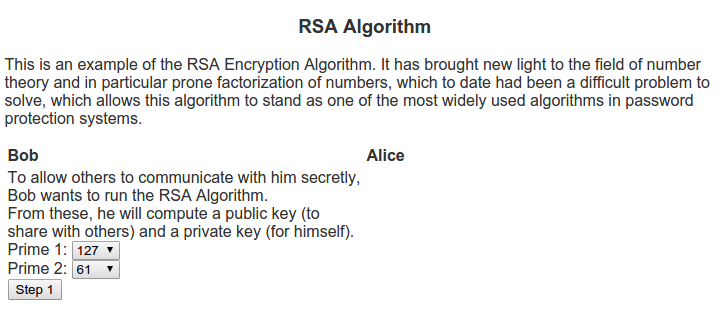I can remember back when I was in school, still deciding whether I wanted to study pure or applied mathematics. One of the common questions I would receive from those in applied mathematical realms would sound like “What’s the point of doing mathematics with no real world applications?”. Generally my response to these questions was about the intrinsic beauty of mathematics, no different from an artist painting not for some desire to be a millionaire, but because of an burning desire to paint. Whether their paintings would one day be on the walls of a Smithsonian museum or sit on their mother’s refrigerator is generally outside of the thought process of the artist. So too, would I argue about the thought process of a pure mathematician.

When I was an undergrad and learned about the RSA algorithm (named for Ron Rivest, Adi Shamir, and Leonard Adleman who discovered the algorithm) it helped me explain this concept a lot better. The algorithm is based on prime numbers and the problem of finding the divisors of a given number. Many mathematicians throughout the ages have written papers on the beauty of prime numbers (see Euclid, Eratosthenes, Fermat, Goldbach, etc). For a large period in time one of the beautiful things about prime numbers was that they were so interesting in themselves. There were questions about how to check if a number is prime, question of patterns in primes, famous conjectures like the Goldbach conjecture and the twin prime conjecture, quick ways of finding prime numbers or numbers that are almost always prime, etc. In short, this was an active area of research that much of the applied world was not using. This all changed in 1977 when Rivest, Shamir and Adleman published the RSA algorithm.

The algorithm is in the area called public key cryptography. These algorithms differ from many of the previous cryptography algorithms, namely symmetric key cryptography. Whereas symmetric key cryptography depends uses the same device (key) to encode as to decode, public key cryptography creates two keys – one for encoding that is generally shared with others, and another for decoding which is kept private. These two keys in generally relate to a mathematical problem that is very difficult to solve.

In my example script for the RSA Algorithm, I show two people who want to communicate, Alice and Bob. Bob wants people to be able to send him messages securely so he decides to use the RSA algorithm. To do this, he first needs to think of two prime numbers, p1 and p2.
From these, he computes the following:
n = p1 * p2

Next, he computes Euler’s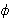function on this n which can be calculated as(n) = (p1 – 1) * (p2 – 1)

Then Bob looks for a number e that is relatively prime to. This is what he will use as the encryption key.

One he has e, he can calculate d, which is the multiplicative inverse of e in
(n).
This means that e * d = 1 (mod(n)).

The public key that will be used for encryption will be the pair (e, n). This is what he posts publicly via his web page for others to communicate with him securely. Bob also has a private key pair (d, n) that he will use to decrypt messages.

Alice sees Bob’s public key and would like to communicate with him. So she uses it to encode a message. The formula she uses to encrypt her message is c = me mod n, where c is the encrypted message. Once Alice encrypts her message, she sends it to Bob.

Bob receives this encoded message and uses the private key (d, n) to decode the message from Alice. The formula to decrypt is m = cd mod n.

For a more illustrative idea of how this algorithm works as well as examples, be sure to visit Script for the RSA Algorithm.

# How To Take Notes in Math Class

I was recently asked by someone how they should be taking notes in math class. I could immediately relate because I once asked this very same question. In both undergrad and grad, I had to ask myself how to take notes because I often would leave class with a bunch of sentence fragments based on what the professor said, but without anything I could use as a study guide. At best, it would be a garbled thing that I could combine with somebody else’s notes and try to make some legible out of it. Generally though, I would just ignore my notes and go to the text book if it was well written, or the library if the text book was not well written.

So how did I get past this? Well, after taking the Set Theory course, I started seeing mathematics as more of a construction job, like building a house. Mathematics is based on proofs which is nothing more than logical reasoning, and logical reasoning is just a series of statements that are either assumptions, definitions, or conclusions drawn from those assumptions based on known facts. The main purpose of classes is to present these “known facts” to the students and help them become more informed mathematicians. So what is a mathematical fact?

There are two important types of facts we generally learn in mathematics. The first gives us a language we can work from – these are our definitions. These are the most important things in a mathematics class because if it is a class on “group theory”, then one of the first definitions will be of a “group,” and not knowing this definition will cause problems when you are trying to prove that something is or is not a group. Similarly, if your class is on solving quadratic equations, then it is very important to be able to define what a “quadratic equation” is. Definitions in mathematics are important because, unlike in an English class, you cannot always derive the meaning of a mathematical term from its usage. Mathematical definitions are very precise and your notes should include this precision.

As a side note, I will state (if not stress) that examples are not definitions. Examples are meant to bring the definition to life, and to help connect the definition to the student, but if you are asked what is a group and respond that “the set of integers mod 7 under the operation of addition is a group”, you’d be incorrect because you gave an example of a group without telling why this is a group. Similarly, if I were to ask you to define a quadratic equation and you said “x^2 + 2x + 1 = 0 is a quadratic equation”, you’d be giving me an example but not a definition. It is important to understand the distinction between examples and definitions because when we understand the definition we can clearly explain why (ala prove) that the given instance is in fact an example.

Some professors will briefly mention a definition and then focus most of their time on examples in an attempt to make the concepts easier to understand. As a student though, it is important to ask the question “what is the definition of _____” because the exams, or the research that follows, or the applications of this concept in real life are not likely to be that same example. If the professor does not write out the definition of whatever concept you are studying, or you are unclear of this definition you should ask questions, go to an online resource, or a text book for supplementary reference.

The second type of fact presented in mathematical classes is the theorem (aka lemma or corollary). A theorem is a statement that is provable by mathematical reasoning. In a classroom setting, theorems will generally be presented in an orderly fashion such that if Theorem 1 is presented before Theorem 2, then Theorem 1 does not require Theorem 2 in order to be proven.

Because theorems are provable statements, it may be tempting to jump directly into the proof, and particularly to only take notes on the proof. I cannot stress against this enough. Before writing the proof, you should make sure to begin the proof with a clear statement of the theorem. This should be a declarative statement that is thus provable. Do not confuse the questions a professor may ask before proving a theorem with the theorem itself. An example of a theorem from group theory is “Any group that has a prime number of elements of elements in that group is cyclic (or can be generated by a single element). Also, do not confuse the nicknames of theorems with the theorem itself. For instance Lagrange’s Theorem says that “the number of elements of any subgroup of a finite group divides the number of elements in the original group.” Remembering this as Lagrange’s Theorem is fine, but it is much more important to remember the declarative statement proved.

I’m sure there are other things people use to take notes in math classes. Feel free to leave a comment sharing some of this advice.